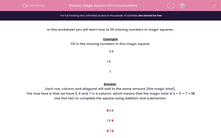# Fill in the Numbers in a Magic Square

In this worksheet, students will use a simple rule to work out the missing numbers in a magic square.Key stage:  KS 2

Curriculum topic:   Maths and Numerical Reasoning

Curriculum subtopic:   Square Numbers

Difficulty level:#### Worksheet Overview

In this activity, we will learn how to fill in missing numbers in magic squares.

Example

Fill in the missing numbers in this magic square.

 3 4 1 5 7

Each row, column and diagonal must add to the same amount (the magic total).

The clue here is that we have 3, 5 and 7 in a column, which means that the magic total is 3 + 5 + 7 = 15

Use this fact to complete the square using addition and subtraction.

 8 3 4 1 5 9 6 7 2

Let's try some ourselves now.

### What is EdPlace?

We're your National Curriculum aligned online education content provider helping each child succeed in English, maths and science from year 1 to GCSE. With an EdPlace account you’ll be able to track and measure progress, helping each child achieve their best. We build confidence and attainment by personalising each child’s learning at a level that suits them.

Get started